# jagshelper-vignette

``library(jagshelper)``

# jagshelper

The goal of `jagshelper` is to streamline Bayesian analysis in JAGS using the `jagsUI` package.
Functions are provided for extracting output in a simpler form, assessing model convergence, and plotting model output. Also included is a function giving a template model in JAGS syntax with the associated `jagsUI` code.

Some of the `jagshelper` functionality is illustrated below, with steps approximately corresponding to those of a typical Bayesian analysis using JAGS.

## Model template

When starting a Bayesian analysis in JAGS from scratch, I can never remember the exact structure for doing so, and sometimes need reminding of basic JAGS model syntax.

The `skeleton()` function prints a JAGS model template to the screen, along with code to simulate a corresponding dataset.

``````library(jagshelper)
skeleton("EXAMPLE")
#>
#> library(jagsUI)
#>
#> # specify model, which is written to an external file
#> cat('model {
#>   for(i in 1:n) {
#>     y[i] ~ dnorm(mu[i], tau)
#>     mu[i] <- b0 + b1*x[i] + a[grp[i]]
#>   }
#>
#>   for(j in 1:ngrp) {
#>     a[j] ~ dnorm(0, tau_a)
#>   }
#>
#>   tau <- pow(sig, -2)
#>   sig ~ dunif(0, 10)
#>   b0 ~ dnorm(0, 0.001)
#>   b1 ~ dnorm(0, 0.001)
#>
#>   tau_a <- pow(sig_a, -2)
#>   sig_a ~ dunif(0, 10)
#> }', file="EXAMPLE_jags")
#>
#>
#> # simulate data to go with the example model
#> n <- 60
#> x <- rnorm(n, sd=3)
#> grp <- sample(1:3, n, replace=T)
#> y <- rnorm(n, mean=grp-x)
#>
#> # bundle data to pass into JAGS
#> EXAMPLE_data <- list(x=x,
#>                  y=y,
#>                  n=length(x),
#>                  grp=as.numeric(as.factor(grp)),
#>                  ngrp=length(unique(grp)))
#>
#> # JAGS controls
#> niter <- 10000
#> ncores <- 3
#>
#> {
#>   tstart <- Sys.time()
#>   print(tstart)
#>   EXAMPLE_jags_out <- jagsUI::jags(model.file="EXAMPLE_jags", data=EXAMPLE_data,
#>                              parameters.to.save=c("b0","b1","sig","a","sig_a"),
#>                              n.chains=ncores, parallel=T, n.iter=niter,
#>                              n.burnin=niter/2, n.thin=niter/2000)
#>   print(Sys.time() - tstart)
#> }``````

## Output summary & Assessing convergence

### A simple model

Having run a model for the first time, it can be useful to see how many parameter nodes have been saved in total, or how many nodes exist for each named parameter. This can aid in deciding the appropriate strategy for assessing convergence: for example, whether trace plots can be feasibly assessed for all parameter nodes in the model, or just a subset.

In the example below, there are relatively few parameters saved and all trace plots can be examined.

``````nparam(asdf_jags_out)  # how many parameters in total
#>  8
nbyname(asdf_jags_out)  # how many parameters (or dimensions) per parameter name
#> \$b0
#>  1
#>
#> \$b1
#>  1
#>
#> \$sig
#>  1
#>
#> \$a
#>  3
#>
#> \$sig_a
#>  1
#>
#> \$deviance
#>  1
tracedens_jags(asdf_jags_out, parmfrow=c(3,3))  # trace plots for all parameters``````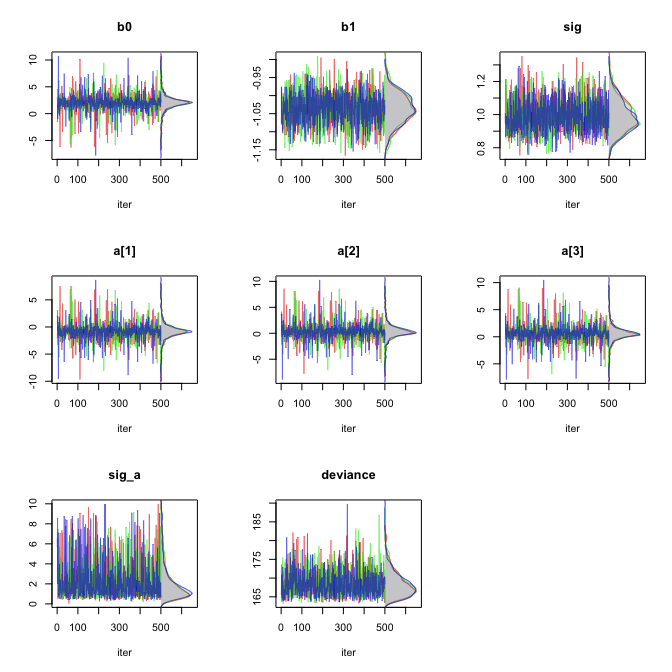``````check_Rhat(asdf_jags_out)  # proportion of Rhats below a threshold of 1.1
#>       b0       b1      sig        a    sig_a deviance
#>        1        1        1        1        1        1
asdf_jags_out\$Rhat  #  Rhat values
#> \$b0
#>  0.999954
#>
#> \$b1
#>  1.00248
#>
#> \$sig
#>  1.00394
#>
#> \$a
#>  1.0002087 0.9995671 1.0000089
#>
#> \$sig_a
#>  1.000986
#>
#> \$deviance
#>  1.001197``````

### A more complex model

In the example below, there are many more parameters saved, and it is perhaps more illustrative to examine the trace plots associated with the least- converged parameter nodes, as measured by `Rhat` value (Gelman & Rubin 1992).

``````nparam(SS_out)  # how many parameters in total
#>  334
nbyname(SS_out)  # how many parameters (or dimensions) per parameter name
#> \$trend
#>  41
#>
#> \$rate
#>  41
#>
#> \$ypp
#>  41
#>
#> \$fit
#>  41
#>
#> \$sig_eps
#>  1
#>
#> \$sig_xi
#>  1
#>
#> \$sig_omega
#>  2
#>
#> \$cycle
#>  41
#>
#> \$cycle_s
#>  41  2
#>
#> \$ar1
#>  41
#>
#> \$phi
#>  1
#>
#> \$deviance
#>  1
traceworstRhat(SS_out, parmfrow=c(3,2))  # trace plots for least-converged nodes``````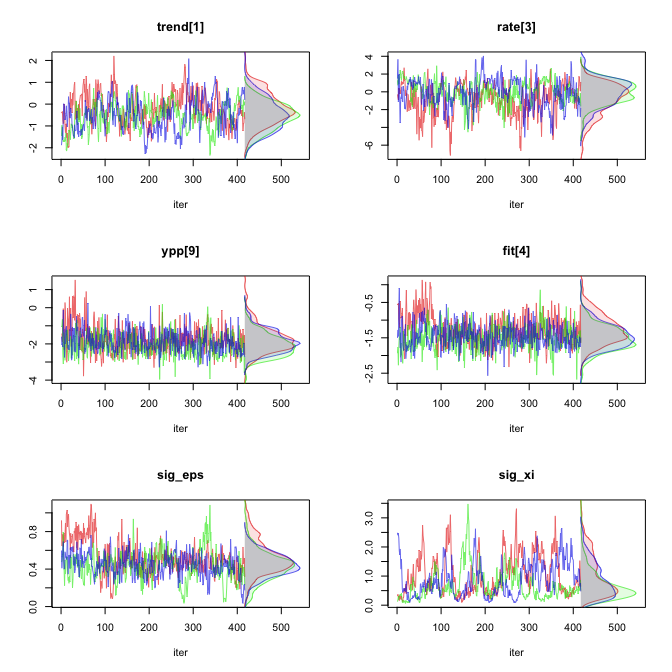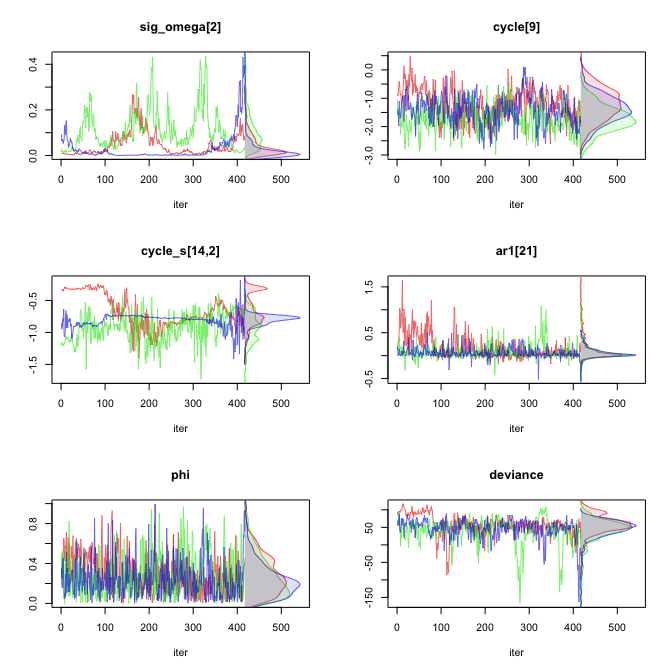``````check_Rhat(SS_out)  # proportion of Rhats below a threshold of 1.1
#>     trend      rate       ypp       fit   sig_eps    sig_xi sig_omega     cycle
#> 1.0000000 1.0000000 1.0000000 0.9268293 1.0000000 1.0000000 0.5000000 0.8780488
#>   cycle_s       ar1       phi  deviance
#> 0.5121951 0.7692308 1.0000000 1.0000000``````
``plotRhats(SS_out)  # plotting Rhat values``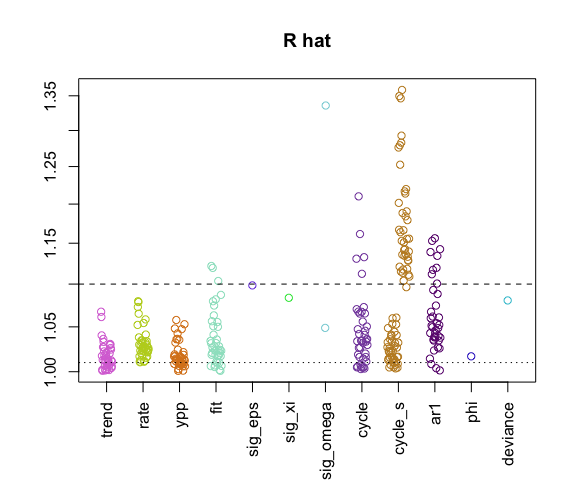• The function `check_neff()` behaves very similarly to `check_Rhat()`, but makes comparisons based on `n.eff` (a crude measure of effective sample size) rather than Gelman-Rubin convergence diagnostic `Rhat`.

• The functions `traceworstRhat()` and `check_Rhat()` both contain an optional `n.eff=` argument. When set to `TRUE`, the functions will compare or plot based on the value of `n.eff` rather than `Rhat`.

• The function `tracedens_jags()` is likely to be the most useful and concise trace plot version. However, if only line trace plots or by-chain kernel density plots are desired (rather than both), older versions `trace_jags()` and `chaindens_jags()` are preserved.

• The function `pairstrace_jags()` gives methods for plotting two-dimensional trace plots, scatter plots, or contour plots, in which each possible pairing of parameter nodes are plotted with respect to one another. In addition to convergence, this may provide a graphical check for correlation between parameter nodes, or problematic posterior surface shapes. An example is shown below.

``pairstrace_jags(asdf_jags_out, p=c("a","sig_a"), points=TRUE, parmfrow=c(3,2))``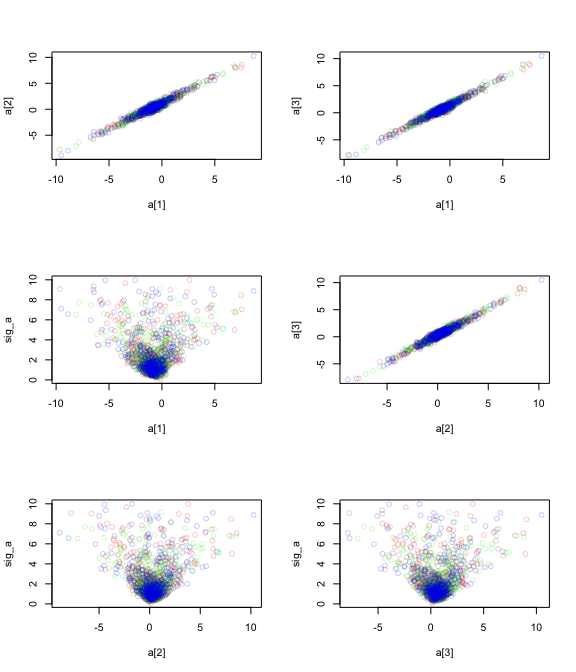• The functions `cor_jags()` and `plotcor_jags()` respectively return and plot correlation matrices of all or a subset of parameters, which may be useful in directly assessing correlation between parameters. In the case of multiple nodes per parameter (as in a vector or array of nodes), the full collection of nodes per parameter name is given one axis tick. This is intended to reduce graphical clutter as well as giving greater visual weight to single parameters. An example is given below.
``plotcor_jags(SS_out, p=c("trend","rate","sig"))``